# CenterNet Pose Estimation Nets Trained onMS-COCO Data

Detect and localize human joints and objects in an image

Released in 2019, this family of models estimates the locations of human joints in an image. In a similar manner to the CenterNet object detection models, these models generate heat maps for each human joint class, and the heat maps are then corrected by the regressed joint offsets. In order to group the predicted keypoints by different human instances, the models also detect entire human bodies separately and parametrize each keypoint by a displacement from the center of the body. Finally, the keypoints regressed from the object centers are aligned with the closest keypoints extracted from the human pose heat maps. Note that CenterNet MobileNetV2 detects only the human instances while ResNet models detect all 80 classes in the MS-COCO dataset.

## Training Set Information

• Microsoft COCO, a dataset for image recognition, segmentation and captioning, consisting of more than three hundred thousand images in 80 different object classes.

## Model Information

•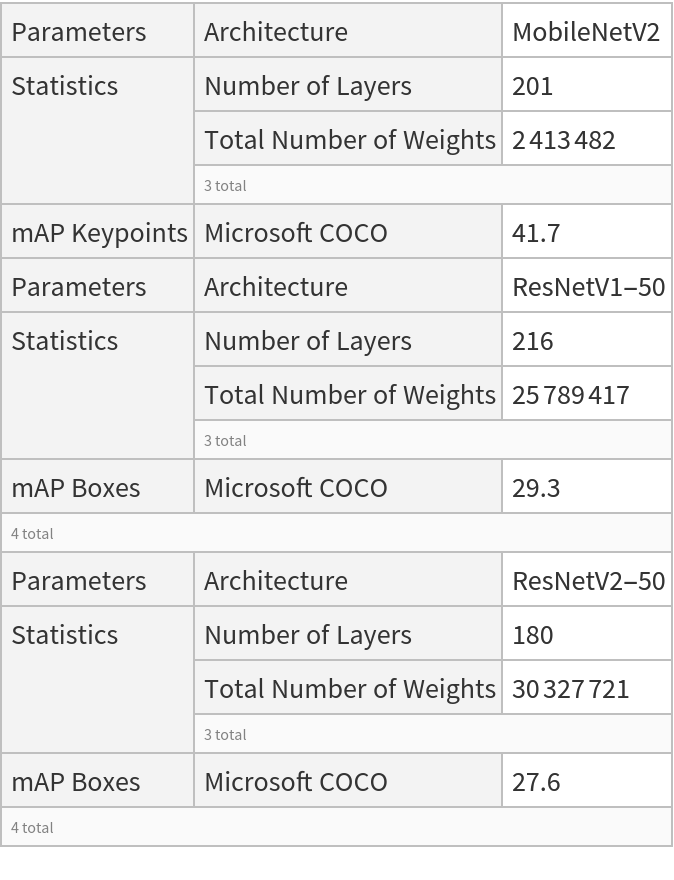## Examples

### Resource retrieval

Get the pre-trained net:

 In:=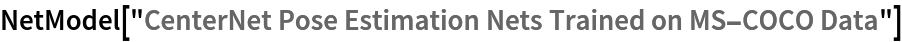Out=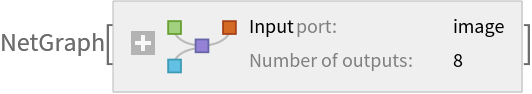### NetModel parameters

This model consists of a family of individual nets, each identified by a specific parameter combination. Inspect the available parameters:

 In:=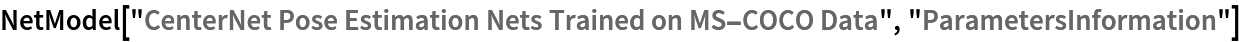Out=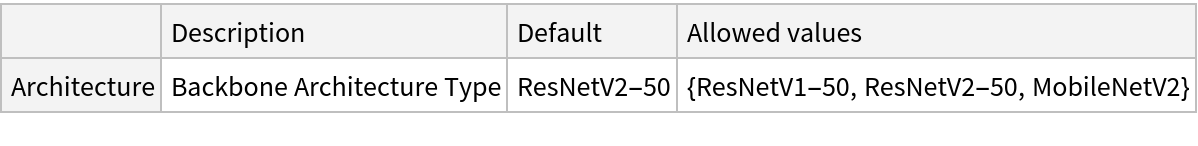Pick a non-default net by specifying the parameters:

 In:=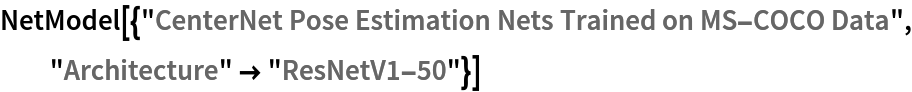Out=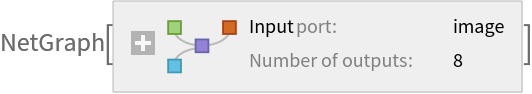Pick a non-default uninitialized net:

 In:=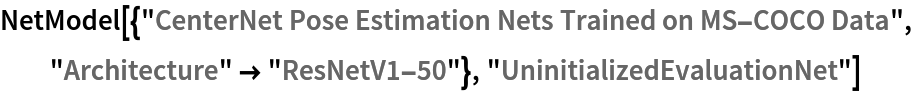Out=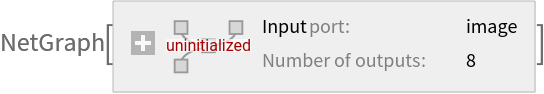### Evaluation function

Define the label list for this model:

 In:=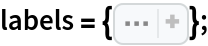Define helper utilities for netevaluate:

 In:=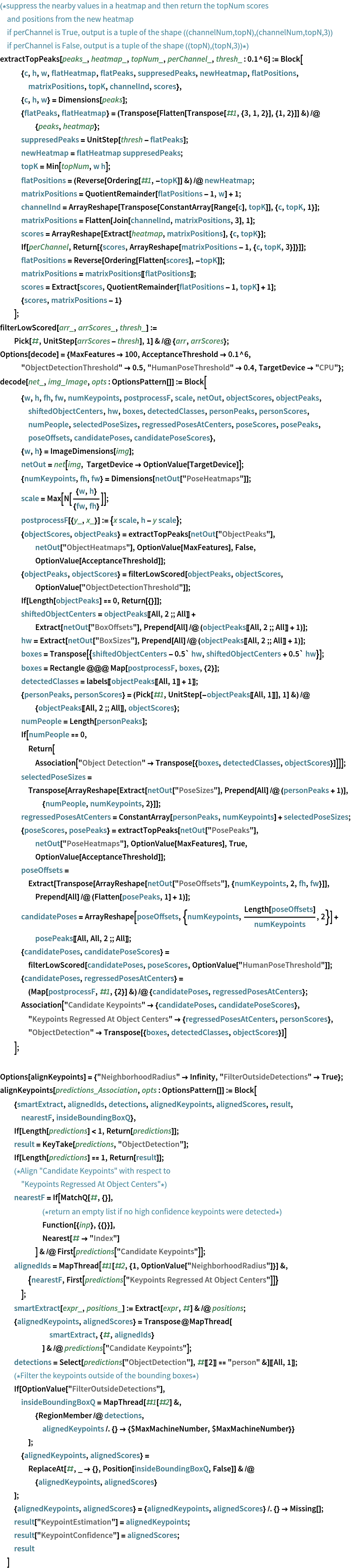Write an evaluation function to estimate the locations of the objects and human keypoints:

 In:=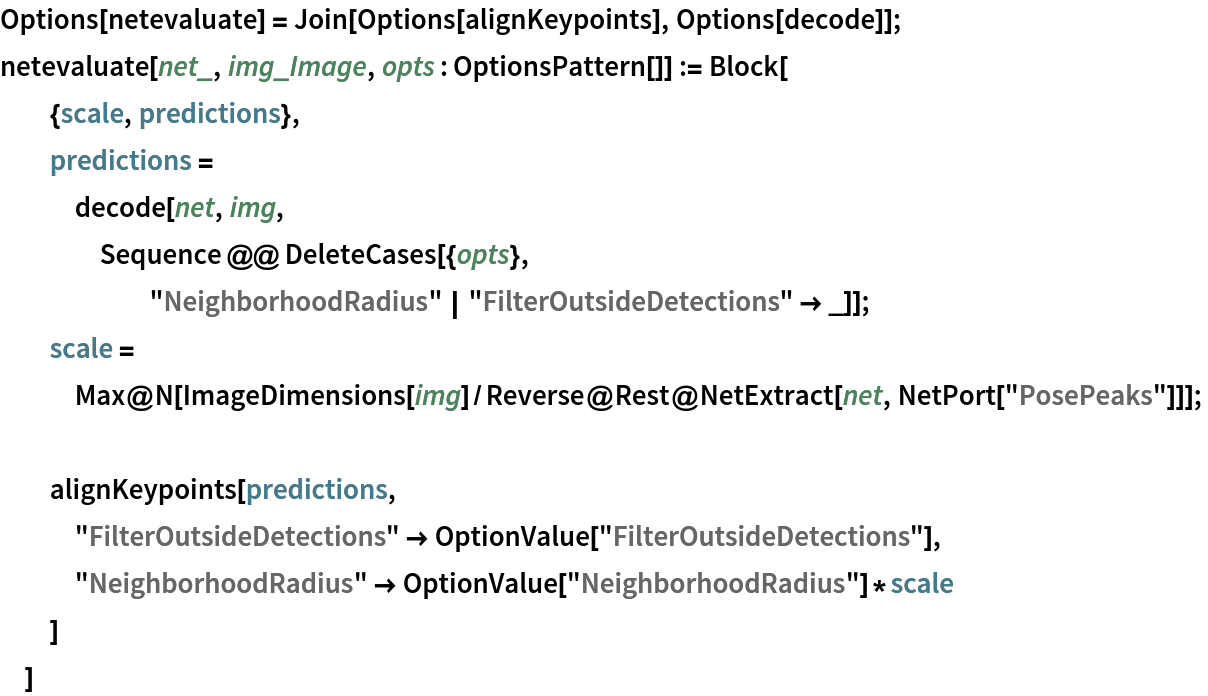### Basic usage

Obtain the detected bounding boxes with their corresponding classes and confidences as well as the locations of human joints for a given image:

 In:=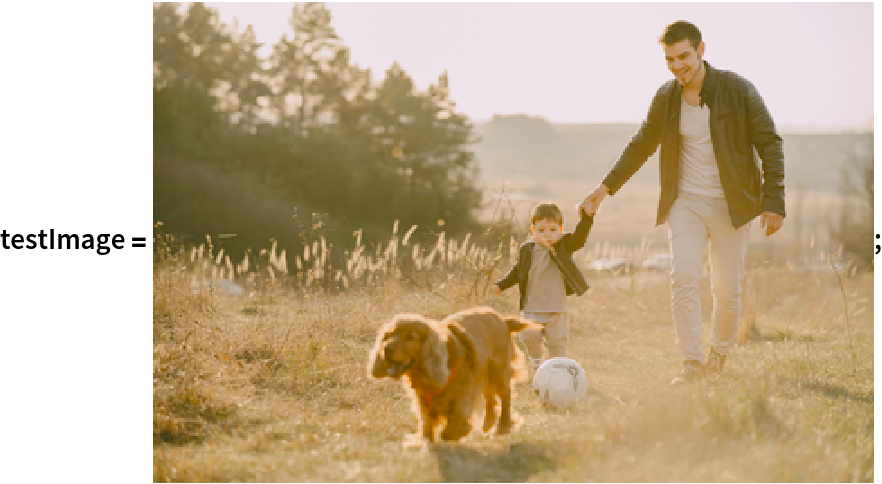In:=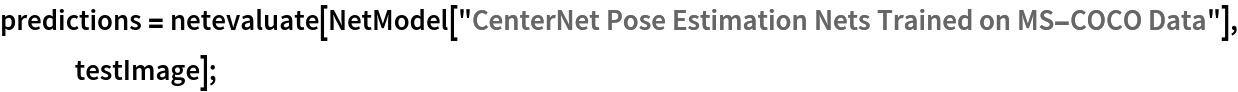Inspect the prediction keys:

 In:=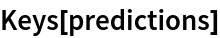Out=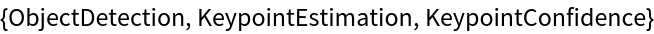The "ObjectDetection" key contains the coordinates of the detected objects as well as its confidences and classes:

 In:=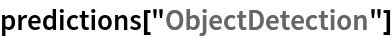Out=Inspect which classes are detected:

 In:=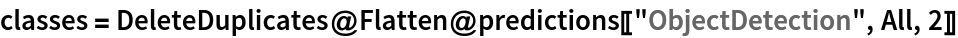Out=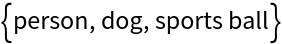The "KeypointEstimation" key contains the locations of top predicted keypoints as well as their confidences for each person:

 In:=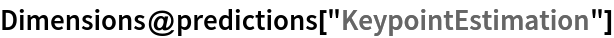Out=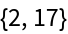Inspect the predicted keypoint locations:

 In:=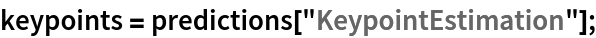Visualize the keypoints:

 In:=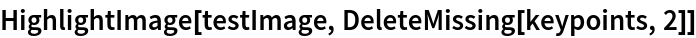Out=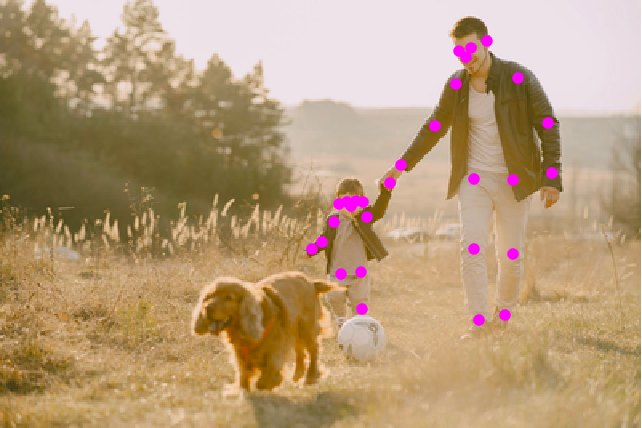Visualize the keypoints grouped by person:

 In:=Out=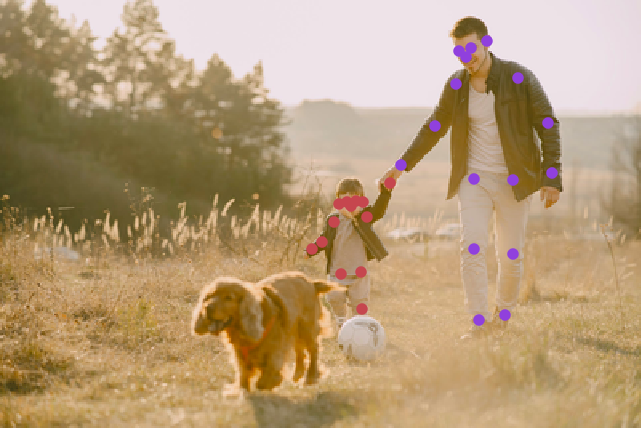Visualize the keypoints grouped by a keypoint type:

 In:=Out=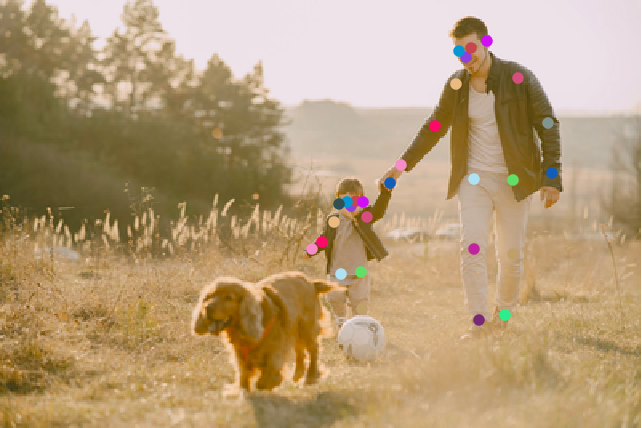Define a function to combine the keypoints into a skeleton shape:

 In:=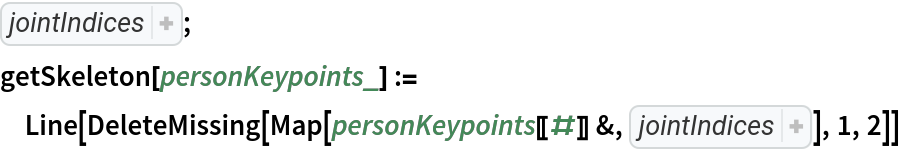Visualize the pose keypoints, object detections and human skeletons:

 In:=Out=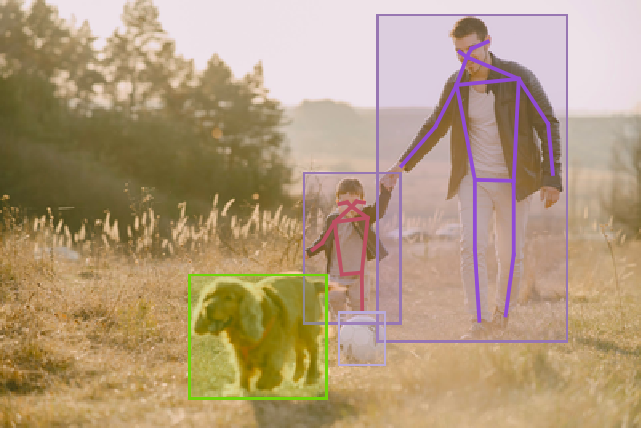In:=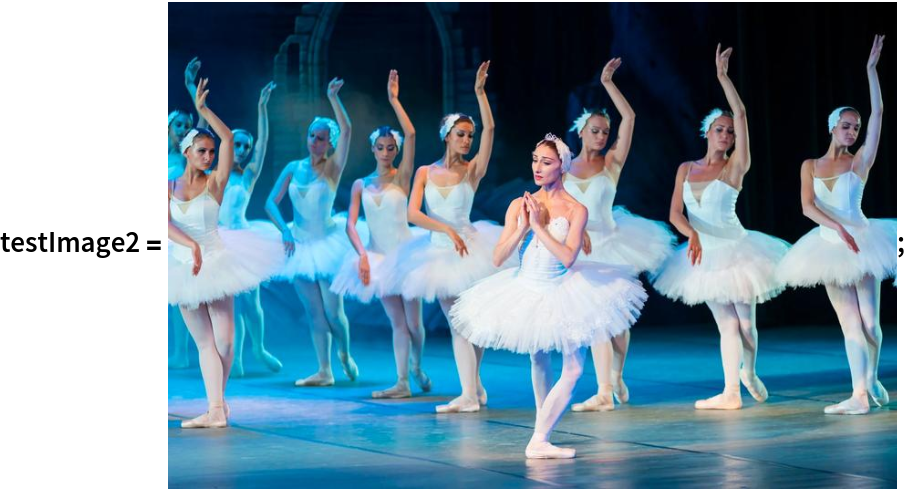Obtain the detected bounding boxes with their corresponding classes and confidences as well as the locations of human joints for a given image:

 In:=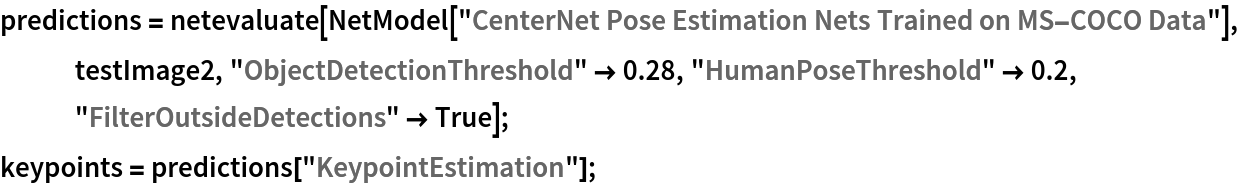Visualize the pose keypoints, object detections and human skeletons. Note that some of the keypoints are misaligned:

 In:=Out=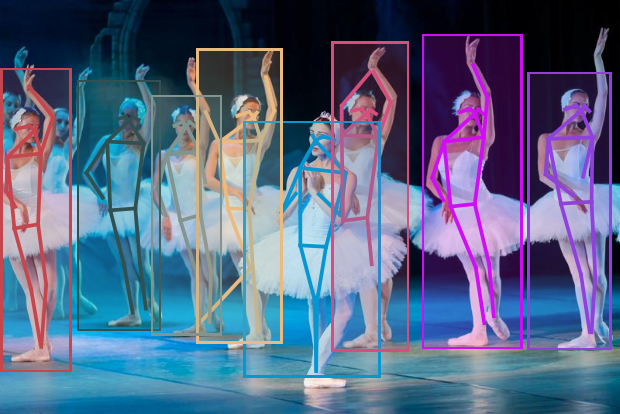Inspect the various effects of a radius defined by an optional parameter "NeighborhoodRadius":

 In:=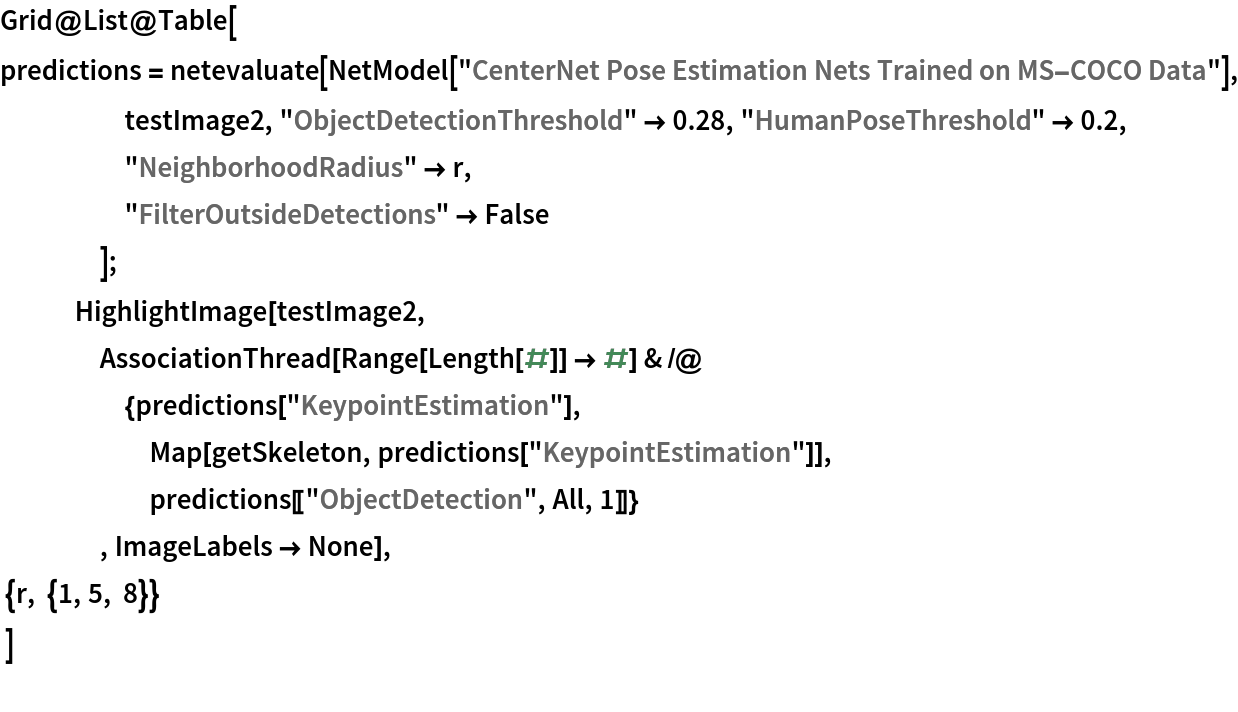Out=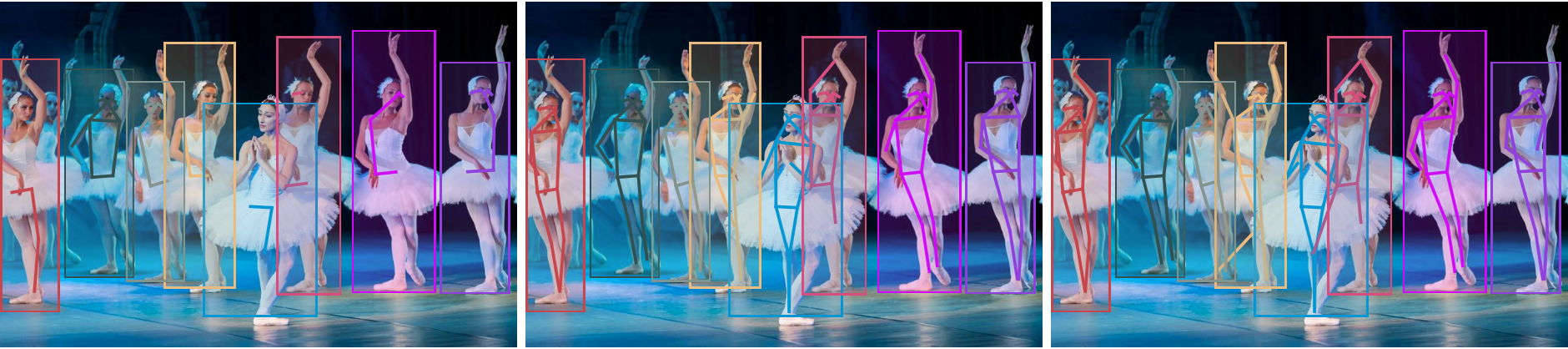### Network object detection result

For the default input size of 512x512, the net produces 128x128 bounding boxes whose centers mostly follow a square grid. For each bounding box, the net produces the box size and the offset of the box center with respect to the square grid:

 In:=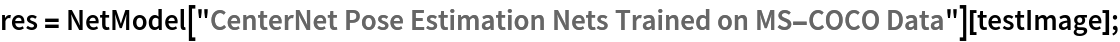In:=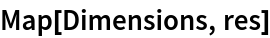Out=Change the coordinate system into a graphics domain:

 In:=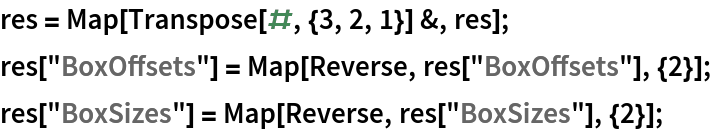Compute and visualize the box center positions:

 In:=Visualize the box center positions. They follow a square grid with offsets:

 In:=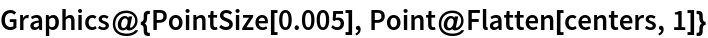Out=Compute the boxes' coordinates:

 In:=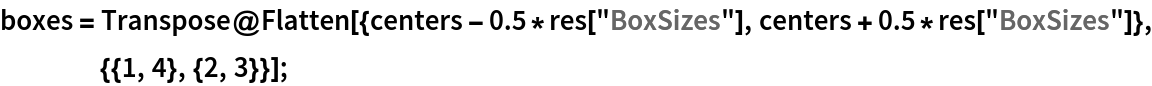In:=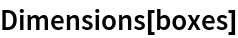Out=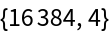Define a function to rescale the box coordinates to the original image size:

 In:=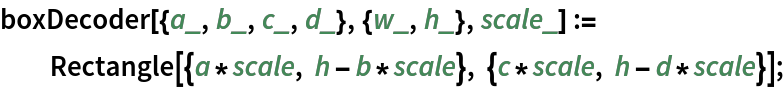Visualize all the boxes predicted by the net scaled by their "objectness" measures:

 In:=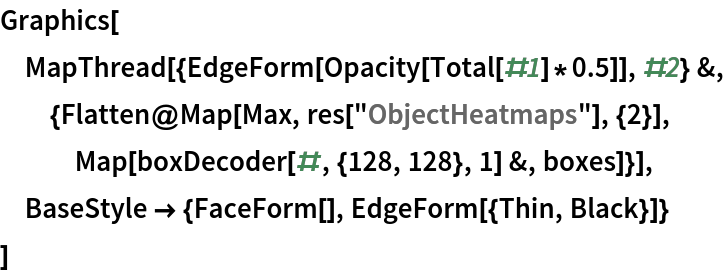Out=Visualize all the boxes scaled by the probability that they contain a dog:

 In:=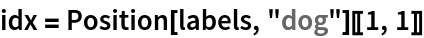Out=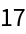In:=Out=Superimpose the cat prediction on top of the scaled input received by the net:

 In:=Out=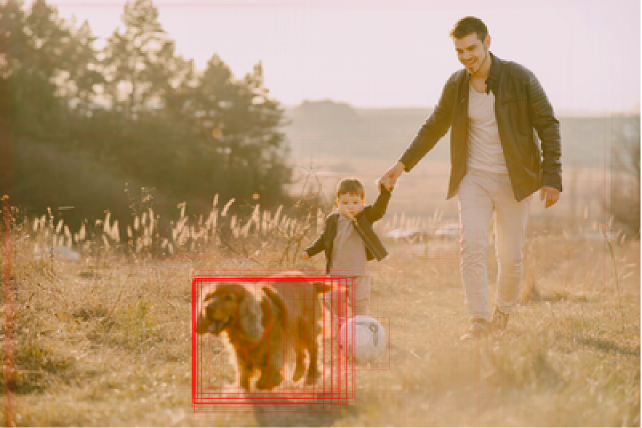### Heat map visualization

 In:=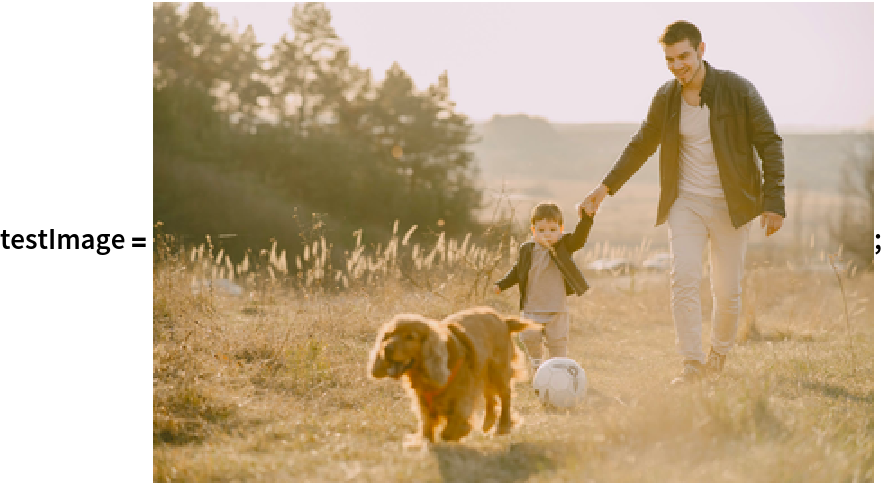Every box is associated to a scalar strength value indicating the likelihood that the patch contains an object:

 In:=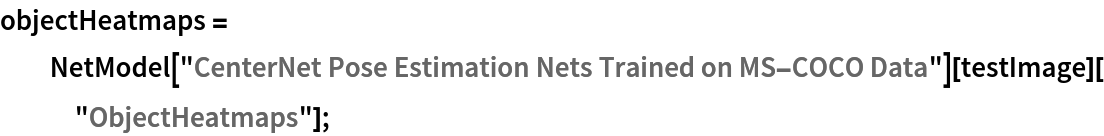In:=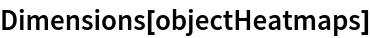Out=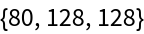The strength of each patch is the maximal element aggregated across all classes. Obtain the strength of each patch:

 In:=Out=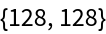Visualize the strength of each patch as a heat map:

 In:=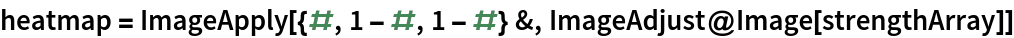Out=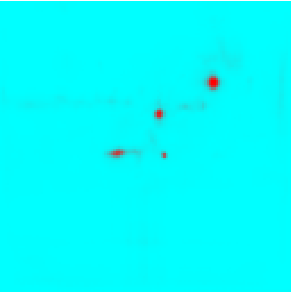Stretch and unpad the heat map to the original image domain:

 In:=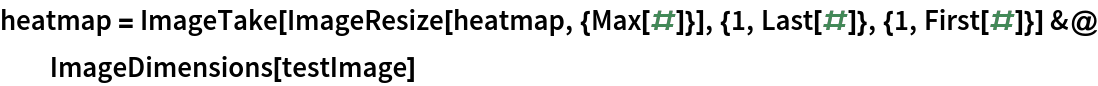Out=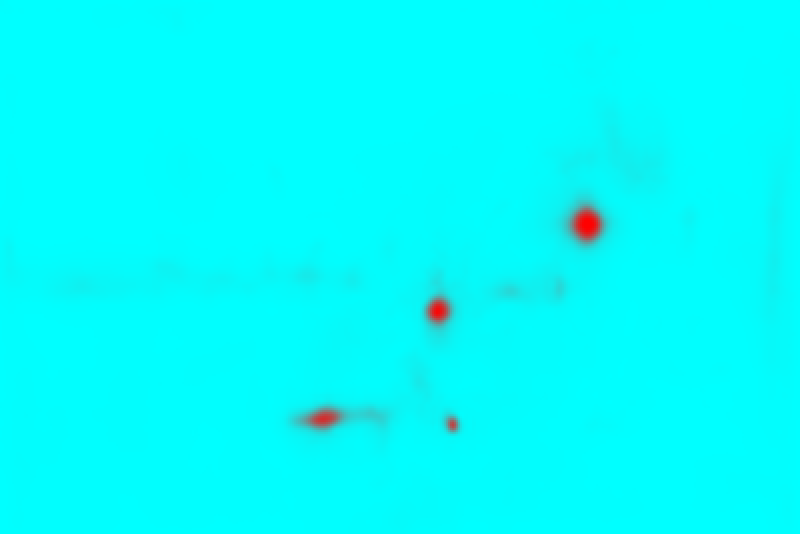Overlay the heat map on the image:

 In:=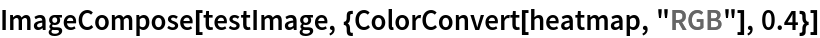Out=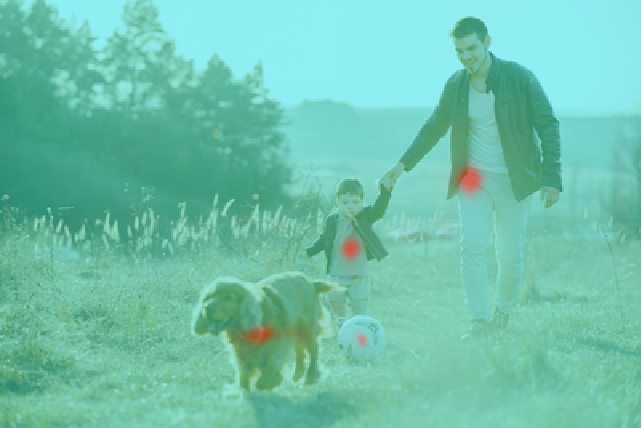Obtain and visualize the strength of each patch for the "dog" class:

 In:=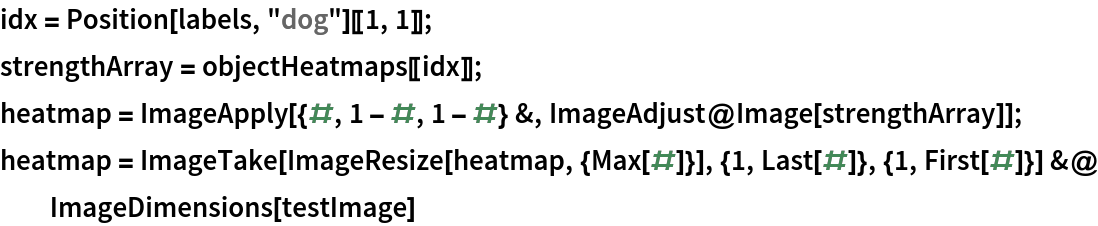Out=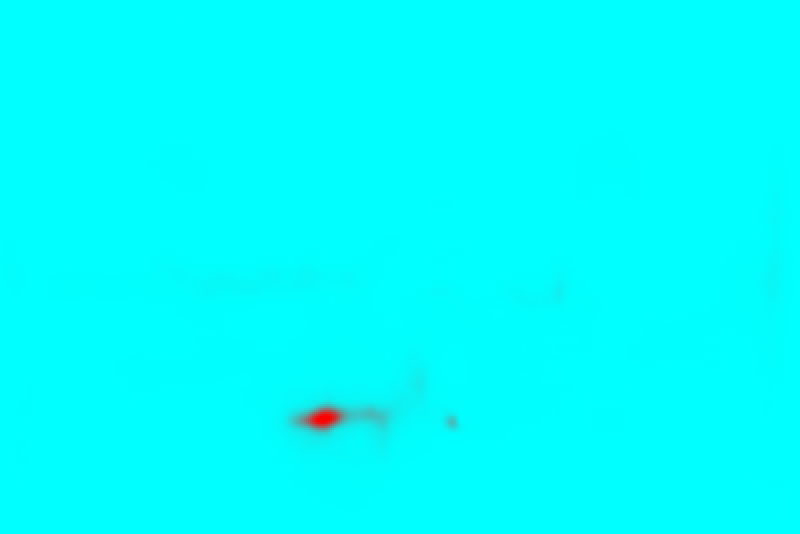Overlay the heat map on the image:

 In:=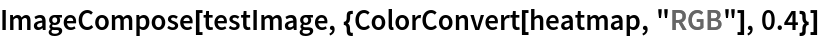Out=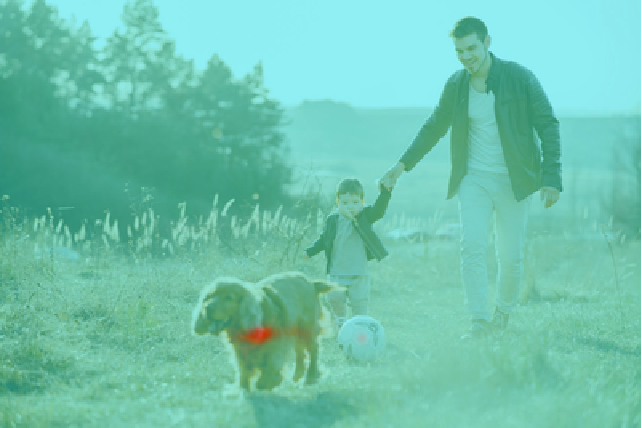Define a general function to visualize a heat map on an image:

 In:=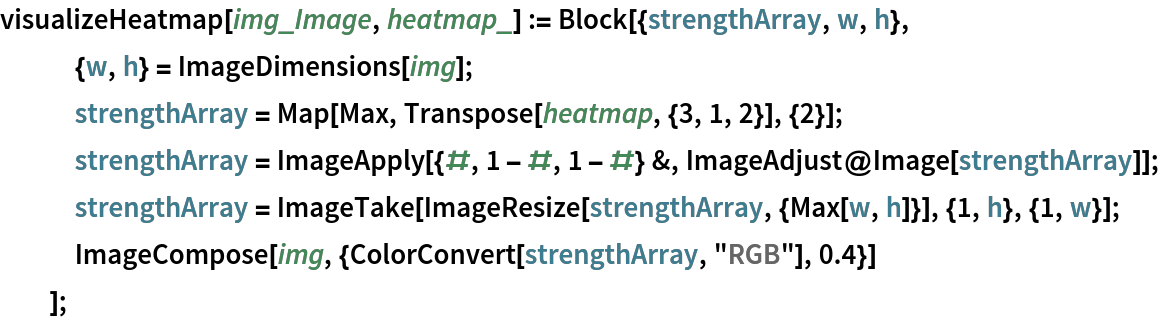In:=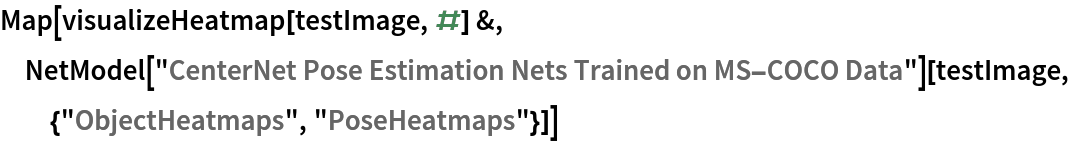Out=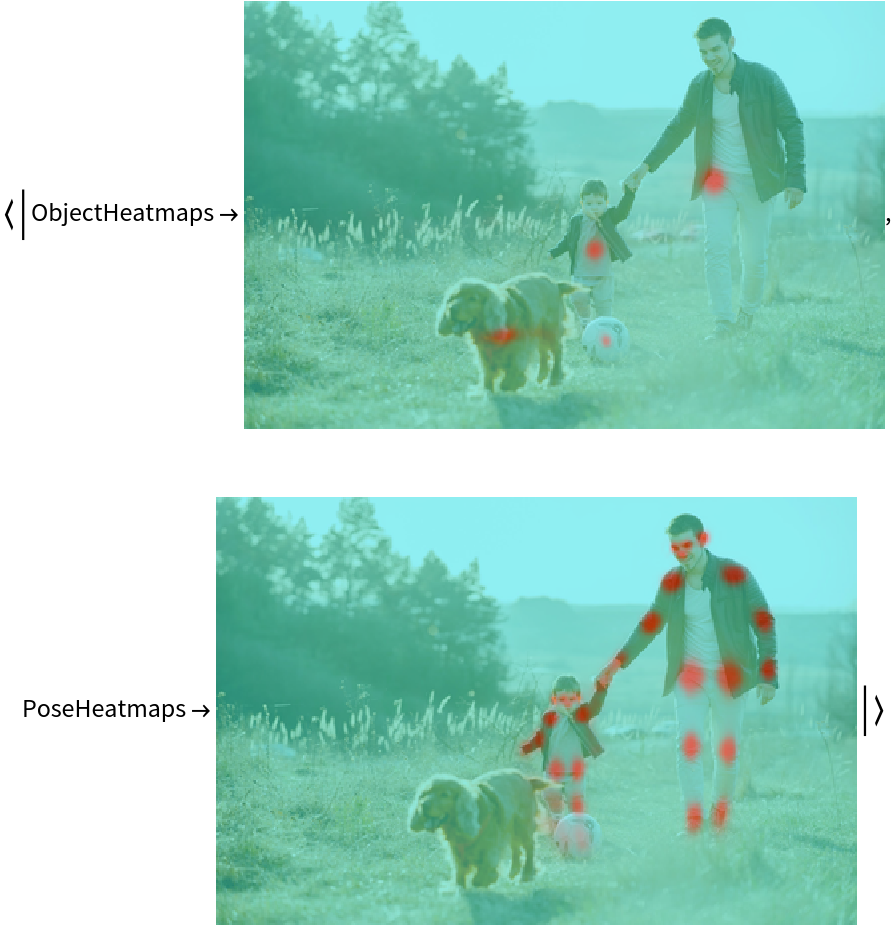Automatic image resizing can be avoided by replacing the NetEncoder. First get the NetEncoder:

 In:=Out=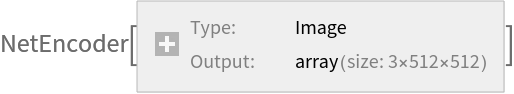Note that the NetEncoder resizes the image by keeping the aspect ratio and then pads the result to have a fixed shape of 512x512. Visualize the output of NetEncoder adjusting for brightness:

 In:=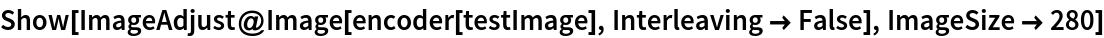Out=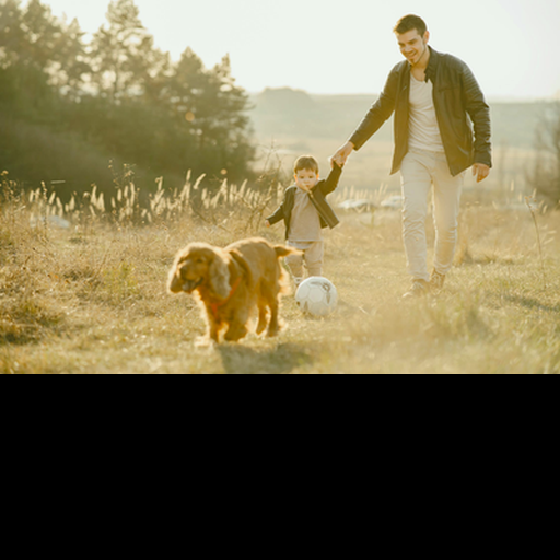Create a new NetEncoder with the desired dimensions:

 In:=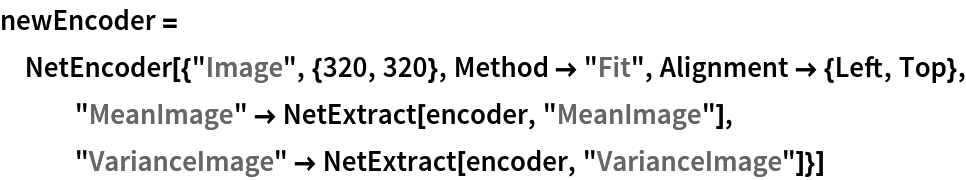Out=Attach the new NetEncoder:

 In:=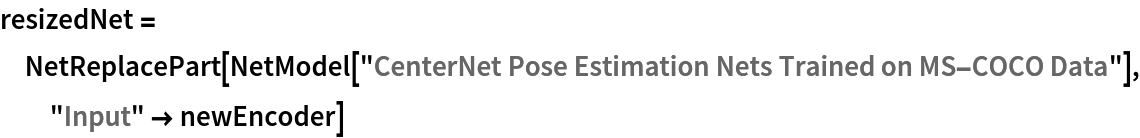Out=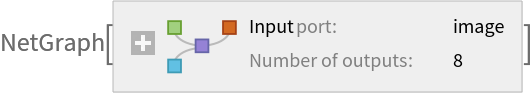Obtain the detected bounding boxes with their corresponding classes and confidences for a given image:

 In:=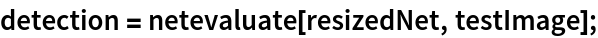Visualize the detection:

 In:=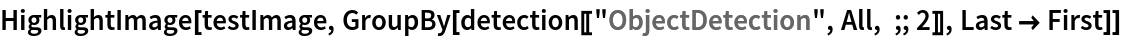Out=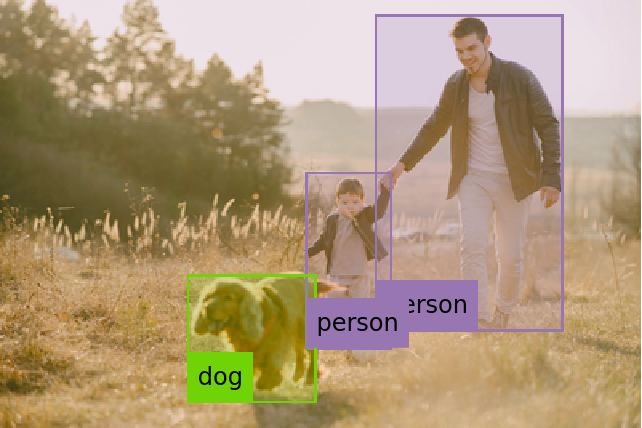Note that even though the localization results and the box confidences are slightly worse compared to the original net, the resized network runs significantly faster:

 In:=Out=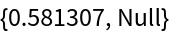In:=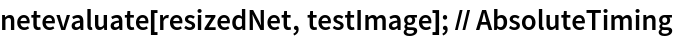Out=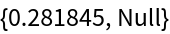### Net information

Inspect the number of parameters of all arrays in the net:

 In:=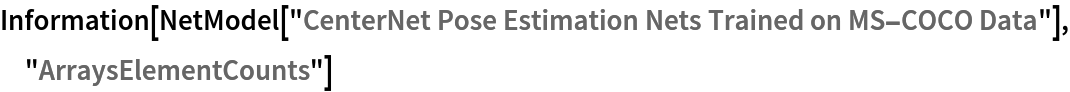Out=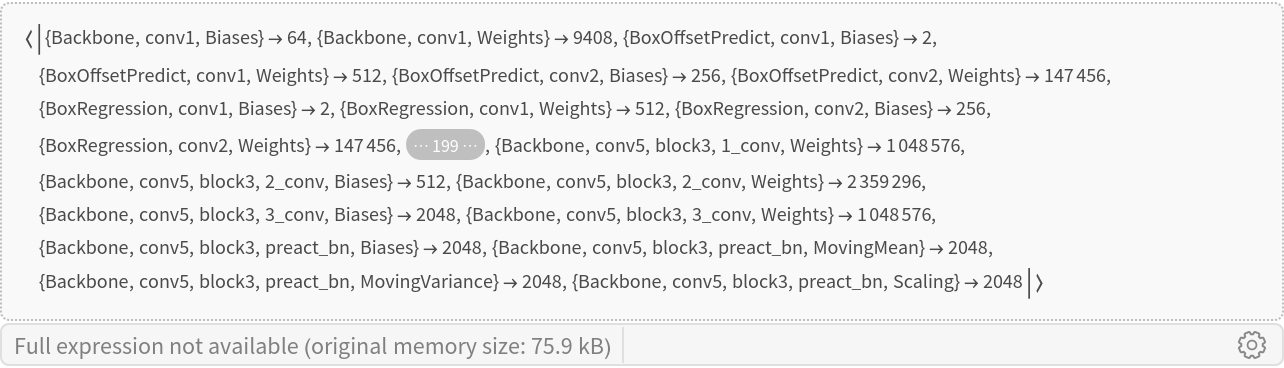Obtain the total number of parameters:

 In:=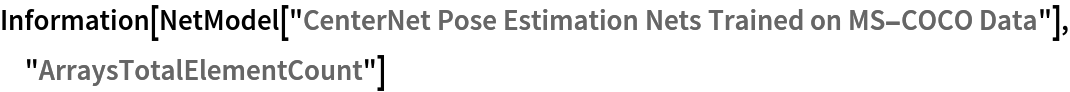Out=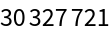Obtain the layer type counts:

 In:=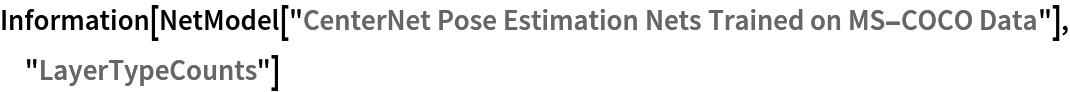Out=Display the summary graphic:

 In:=Out=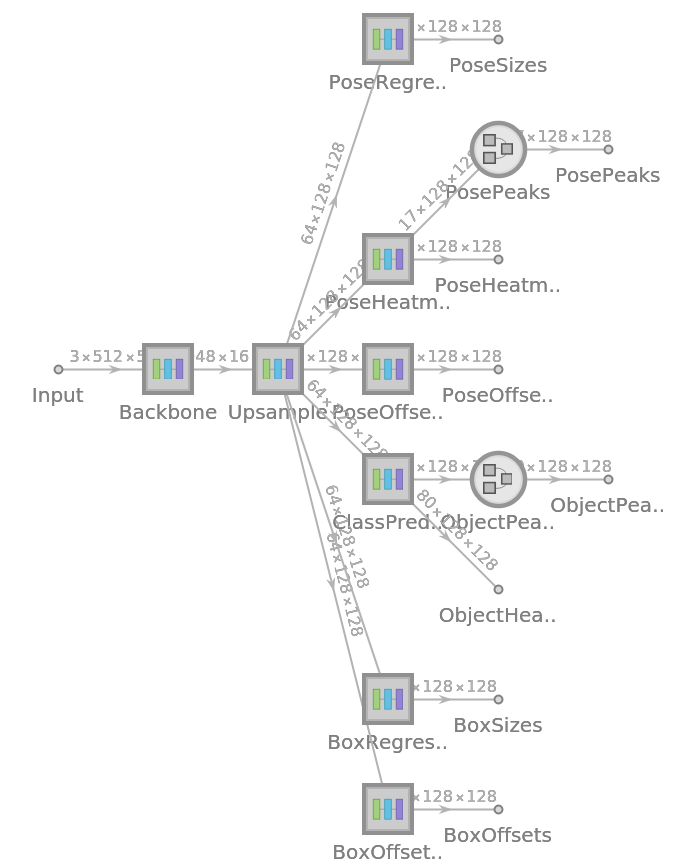## Reference

• X. Zhou, D. Wang, P. Krähenbühl, "Objects as Points," arXiv:1904.07850 (2019)